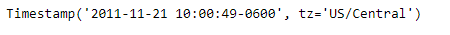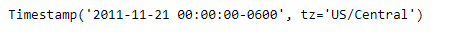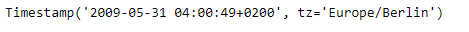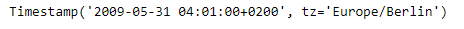# Python | Pandas Timestamp.round

Python is a great language for doing data analysis, primarily because of the fantastic ecosystem of data-centric python packages. Pandas is one of those packages and makes importing and analyzing data much easier.

Pandas` Timestamp.round()` function is used to round the given Timestamp to the specified resolution.

Syntax :Timestamp.round()

Parameters :
freq : a freq string indicating the rounding resolution

Return : a new Timestamp rounded to the given resolution of freq

Example #1: Use `Timestamp.round()` function to round the given Timestamp to Daily time series frequency.

 `# importing pandas as pd ` `import` `pandas as pd ` ` `  `# Create the Timestamp object ` `ts ``=` `pd.Timestamp(year ``=` `2011``,  month ``=` `11``, day ``=` `21``, ` `                  ``hour ``=` `10``, second ``=` `49``, tz ``=` `'US/Central'``) ` ` `  `# Print the Timestamp object ` `print``(ts) `

Output :Now we will use the `Timestamp.round()` function to round the given Timestamp object to Daily frequency.

 `# round to daily frequency ` `ts.``round``(freq ``=` `'D'``) `

Output :As we can see in the output, the `Timestamp.round()` function has returned a Timestamp object having the time series frequency rounded to the desired resolution.

Example #2: Use `Timestamp.round()` function to round the given Timestamp to minutely time series frequency.

 `# importing pandas as pd ` `import` `pandas as pd ` ` `  `# Create the Timestamp object ` `ts ``=` `pd.Timestamp(year ``=` `2009``, month ``=` `5``, day ``=` `31``, ` `       ``hour ``=` `4``, second ``=` `49``, tz ``=` `'Europe/Berlin'``) ` ` `  `# Print the Timestamp object ` `print``(ts) `

Output :Now we will use the `Timestamp.round()` function to round the given Timestamp object to minutely frequency.

 `# round to minutely frequency ` `ts.``round``(freq ``=` `'T'``) `

Output :As we can see in the output, the `Timestamp.round()` function has returned a Timestamp object having the time series frequency rounded to the desired resolution.

My Personal Notes arrow_drop_upCheck out this Author's contributed articles.

If you like GeeksforGeeks and would like to contribute, you can also write an article using contribute.geeksforgeeks.org or mail your article to contribute@geeksforgeeks.org. See your article appearing on the GeeksforGeeks main page and help other Geeks.

Please Improve this article if you find anything incorrect by clicking on the "Improve Article" button below.

Article Tags :

Be the First to upvote.

Please write to us at contribute@geeksforgeeks.org to report any issue with the above content.